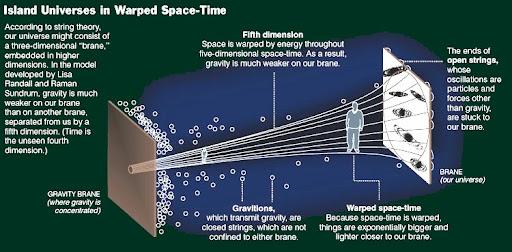# Why does the force of gravity get weaker as it travels through the dimensions?

Some theories predict that the graviton exists in a dimension that we of course can't see, and that is why the force of gravity is so weak. Because by the time gravity has got from the dimension in which the graviton exists to our dimension it has lost much of its strength.

For a better understanding of this theory, I think this might help:Why does the force lose strength as is travels through the other dimensions?

• Hi Olly - are you referring to an existing theory of quantum gravity that offers this explanation? If so, it might help to include a link or two so people can read more about it. (Also it's better to start your question with "Some theories predict that..." rather than "I believe that..." so that it's clear you're talking about accepted science, not trying to promote an idea you made up.) – David Z Apr 29 '12 at 21:16

This is nearly a duplicate of large-extra dimensions questions, you can see the answer here. The theories that predict that gravity is weak because it has extra dimensions are the large extra dimensions theories.

The reason gravity gets weaker is that gravity obeys a version of Gauss's law, which states that the outward gravitational field integrated over a large surface is equal to the mass inside (up to pressure corrections--- this is the Newtonian gravity, but the Einstein gravity is qualitatively identical in its scaling).

If you have a sphere of radius R, it's volume goes like $R^d$ by dimensional analysis, where d is the number of dimensions. The surface area goes like $R^{d-1}$ because it's the derivative of the volume with respect to the radius (the surface area is the volume enclosed by two spheres of infinitesimally different radius divided by the infinitesimal radial difference). So the total gravitational field times $R^{d-1}$ is equal to the mass, so that the gravitational field falls off as $R^{d-1}$ where d is the number of spatial dimensions. For 3 dimensions, it falls off as $R^2$, and this is Newton's universal gravitation.

In higher dimensions, gravity falls off faster. So if there are extra dimensions, and only gravity can propagate in these dimensions, gravity gets weaker quickly until you reach the scale where the extra dimensions are curled up. This means that gravity is weaker than other forces to the extent that the extra dimensions are large.

This type of theory must lower the Planck scale tremendously in order for this to be the dominant explanation of gravity's weakness, and in these circumstances, it runs into problems with non-renormalizable corrections, which should have already been observed. This is discussed in the linked answer.

Within standard string theory however, a miniature version of this mechanism allows gravity and the other forces to unify at a slightly lower energy scale than the Planck scale, nearer to the GUT scale, because the size of the extra dimensions is seen to be non zero around there. Lowering the Planck scale by one or two orders of magnitude in this was is not controversial, and was suggested by Witten in the 1980s, long before large extra dimensions were proposed.

Why gravity gets weak as it "arrives" at our weak brane can be explained by looking at the warped AdS space given by

$ds^2 = e^{-2\phi}\eta_{\mu\nu}\, dx^{\mu} dx^{\nu} + d\phi^2$

The flat Minkowski metric $\eta_{\mu\nu}$ is rescaled by the exponential of the 5th dimension $\phi$. Proper distance and time therefore depend on the position in the 5th dimension given by the value of $\phi$. Relating this to the picture in the question, our universe (the weak brane) sits at the maximal value $\phi_{max}$. This means that proper distance and time are short. The Planck brane where gravity is concentrated is found at the minimum value $\phi_{min}$, leading proper distance and time to be huge there.

In summary, most of the volume of the spacetime described by this model is concentrated near the Planck brane. This leads to the effect that the "wave function" of the gravitons (which can escape into the extra dimension) is concentrated near the Planck brane, such that they only weakly overlap and interact with the "wave functions" of the standard model stuff (that is stuck on our weak brane). Gravity is therefore much weaker than the other forces.

I have stolen this again from Lumo ;-P ...; it can be read in more detail in the subsection with the title Randall-Sundrum introduction in this article.